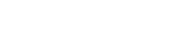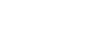# Mathematics Questions and Answers - Form 3 Term 1 Opener Exams 2021 Featured

1. Simplify:                                           ( 4marks )2. Solve for x in the equation.
27x x 3(2x−2) = 9(x +2)                       (3marks)
3. The size of each interior angle of a regular polygon is five times the size of the exterior angle. Find the number of sides of the polygon.                       ( 3 marks )
4. The dimensions of a brick are 2cm x 3.4cm x 6.42cm. Find the percentage error in the calculation of its area.                  (3marks)
5. Masses of three babies was stated as a=12.7kg, b=9.8 kg and c=3.20kg. find the relative error in the following expressions:
1. a+b−c                 (3mks)
2. c÷ab             (3mks)
6. Find the relative error in using 0.3 as the estimate of 1/3.     (2mks)
7. Find the length of AC of triangle ABC in which AB=5cm, <ABC=151° and <BCA=13°. (3mks)
8. In a triangle LMN, <L=81°, n=4.3cm and m=3.5cm. Calculate
1. Length l                   (2marks)
2. Angles M and N                (3marks)9. In triangle ABC, <B=61º, and b = 5.3cm. find the radius of the circle passing through the vertices A,B and C       (3marks)
10. The table below shows height of 50 students
 Height (cm) Frequency 140 – 144 145 – 149 150 – 154 155 – 159 160 – 164 3 16 20 10 1

1. State the modal class        ( 1 mark)
2. Calculate the median height.         ( 3 marks )
11. Use completing square method to solve for X in.      (3marks)
½ x25/2x + 1 =0
12.
1. Complete the table below for the function y = 6 + x – x2.         ( 2 marks )
 x −4 −3 −2 −1 0 1 2 3 4 5 y −14 6 4 −6
2. On the grid provided below, draw the graph of y = 6 + x – x2 for −4 ≤ x ≤ 5.      (3marks )
3. On the same axes draw the graph of y = 2 – 2x.       (2marks )
4. From the graphs, find the values of x which satisfy the simultaneous equations.
y = 6 + x – x2
y = 2 – 2x.                          ( 1 marks )
5. Write down and simplify a quadratic equation in the form ax2 + bx + c = 0 which is satisfied by the values of x where the two graphs intersect.               (2 marks )## Marking Scheme

1. 3/5 x 60 – 8/3 x 3/2                              M1 simplification of numerator
45/8 x 16/95/4 x 24/5 + 14/5 x 10/7
36 – 4                                            M1 simplification of denominator
10 – 6 + 4
32/8 = 4

2. 33x x 3(2x – 2) = 32(x + 2 )         M1 expressing in index form
3x + 2x – 2 = 2x + 4                M1 relating index
3x = 6
x = 2
3. Exterior < = 360
n
Interior < = 180 - 360
n
180 – 360 = 5 ( 360/n )             M1
n
180n – 360 = 1800
n          n              M1
180n = 2160
n = 12             A1
4. The dimensions of a brick are 2cm x 3.4cm x 6.42cm. Find the percentage error in the calculation of its area.      (3 mks)
Relative error = 0.5/2 + 0.05/3.4 + 0.005/6.42 = 0.26548 .......m1
Working Product = 2 x 3.4 x 6.42 =43.656.............m1
Percentage error = 0.26548 x 100 = 0.608%............A1
43.656
5. Masses of three babies was stated as a=12.7kg, b=9.8 kg and c=3.20kg. find the relative error in the following expressions:
1. a+b-c (3mks)
Absolute error = 0.05 + 0.05 + 0.005 = 0.105.......m1
Working (a+b-c)= 12.7+9.8 −3.2 = 19.3.............m1
Relative error = 0.105 = 0.005440..............A1
19.3
2. c÷ab (3mks)
Relative error = 0.05 + 0.05 + 0.005 = 0.01060
12.7      9.8       3.2
6. Find the relative error in using 0.3 as the estimate of 1/3.      (2mks)
Absolute error = 1/33/10 =1/30..................m1
Relative error = 1/30 ÷ 1/3 = 1/10...................m1
7. Find the length of AC of triangle ABC in which AB=5cm, <ABC=151° and <BCA=13°. (3mks)5     =     AC
Sin 13      Sin 151      m1
AC =5Sin 151
Sin 13              m1
=10.78cm             A1
8. In a triangle LMN, <L=81°, n=4.3cm and m=3.5cm. Calculate
1. Length l       (2marks)L2 = 4.32 + 3.52 – 2 x 4.3 x 3.5Cos 81°         M2
L = 5.10 cm
2. Angles M and N    (3marks)
5.1   =   4.3
Sin 81     Sin N
Sin N = 4.3Sin81
5.1
Sin N = 0.8328               M2
N = 56.38°
M = 180 – (81+56.38) = 42.62°          A1
9. In triangle ABC, <B=61°, and b = 5.3cm. find the radius of the circle passing through the vertices A,B and C      (3marks)
5.3    =2R
Sin 61
R =     5.3
2 Sin 61°
R = 3.03 cm
10.
1. Modal class 150 – 154                    B1

2.  Class f cf 140 – 144  145 – 149 150 – 154 155 - 159 160 - 164 3 16 20 10 1 3 19 39 49 50
B1 C.F
M ==149.5 + (25 – 19) x 5
20                  M1

= 151
11. Use completing square method to solve for X in.
½ x25/2x + 1 =0       (   3marks)
x2 – 5x + 2 =0
x2 – 5x + C = −2 + C
x2 – 5x + (5/2)2 = −2 + 6.25
(x – 5/2)2 = 4.25
X – 2.5 = ±2.061
X = ±2.061 + 2.5
X= 2.561 or 0.439
12.

1.  x −4 −3 −2 −1 0 1 2 3 4 5 y −14 −6 0 4 6 6 4 0 −6 −14
B2 b1 for 4 ✓values
2. Graph

3.4. x = −1 or x = 4            B1
5. (x + 1 ) ( x – 4) = 0             M1
x2 – 4x + x – 4 = 0
x2 – 3x – 4 =0                       A1

• ✔ To read offline at any time.
• ✔ To Print at your convenience
• ✔ Share Easily with Friends / Students